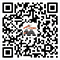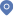Activities
Quantum algorithms for systems of linear equations and differential equationsHome - Activities
Reporter：
Dr. Dong An, Joint Center for Quantum Information and Computer Science, University of Maryland
Inviter：
Xin Liu, Professor
Subject：
Quantum algorithms for systems of linear equations and differential equations
Time and place：
9:00-11:00 March 30 (Thursday), Tencent Meeting: 360-856-258
Abstract：

Solving systems of linear equations and differential equations are fundamental tasks that underlie many areas of science and technology. While classical algorithms typically take time at least proportional to the number of variables, a quantum computer with suitable input access can produce a quantum state that encodes the problem solution with logarithmic complexity, and thus can potentially achieve an exponential speedup over a classical computer. In this talk, we explore the power of quantum algorithms for linear systems and differential equations. For systems of linear equations, we design an efficient quantum algorithm based on time-optimal discrete adiabatic evolution and the eigenvector filtering technique. Our algorithm achieves optimal complexity that is linear in the condition number and logarithmic in the error. For differential equations, we propose a simple method for simulating a general class of non-unitary dynamics as a linear combination of Hamiltonian simulation problems. Our method achieves optimal cost in terms of state preparation and can be applied for open quantum dynamics simulation with the complex absorbing potential.